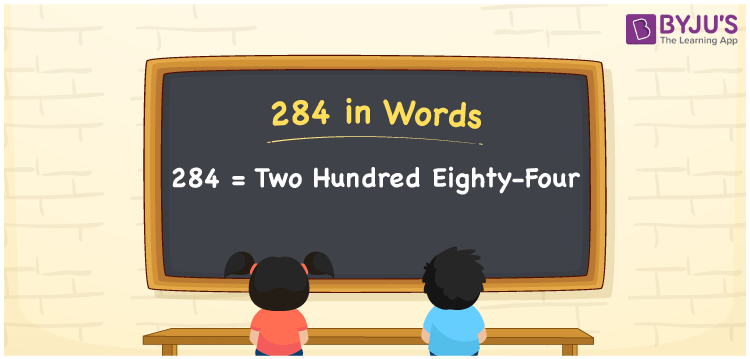# 284 in Words

We can write 284 in words as Two hundred eighty-four. Alternatively, we can say that Two hundred eighty-four is the word form or number name for 284. It is easy to convert the number 284 into word form with the help of a place value chart. Let’s learn how to convert the cardinal number 284 into words and some facts about 284 here in this article.

 284 in words Two hundred eighty-four Two hundred eighty-four in Numbers 284

## 284 in English Words

Generally, we write numbers in words using the English alphabet. So, we spell 284 in English words as “Two hundred eighty-four”.## How to Write 284 in Words?

In this section, you will understand how to convert the number 284 into words using a place value chart with three columns, which is given below.

 Hundreds Tens Ones 2 8 4

Here, ones = 4, tens = 8, hundreds = 2

Let us expand these numbers based on their place values.

2 × Hundred + 8 × Ten + 4 × One

= 2 × 100 + 8 × 10 + 4 × 1

= 200 + 80 + 4

= Two hundred + Eighty + Four

= Two hundred eighty-four

Therefore, 284 in words = Two hundred eighty-four

As we know, 284 is a natural number that precedes 285 and succeeds 283.

284 in words – Two hundred eighty-four

Is 284 an odd number? – No

Is 284 an even number? – Yes

Is 284 a prime number? – No

Is 284 a composite number? – Yes

Is 284 a perfect square number? – No

Is 284 a perfect cube number? – No

## Frequently Asked Questions on 284 in Words

Q1

### How do I spell 284 in words?

We can spell the number 284 in English words as Two hundred eighty-four.
Q2

### Write the value of 33 + 284 in words.

33 + 284 = 317 Therefore, the value of 33 + 284, i.e., 317 in words is written as Three hundred and seventeen.
Q3

### How to write Rs. 284 in words on a cheque?

On a cheque, we can write Rs. 284 in words as “Two hundred eighty-four rupees only”.